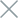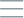• Home
• Tags
• Series
•# TypeScript Syntax and Data Types: An In-depth Guide

## Variable Declaration

To declare variables in `TypeScript`, we use the `let` or `const` keywords.

For example: `let num: number = 10;` or `const message: string = "Hello";`

## `Primitive Data Types`

`TypeScript` supports `primitive data types` such as `number`, `string`, `boolean`, `null`, and `undefined`.

For example: `let age: number = 25;`, `let name: string = "John";`, `let isActive: boolean = true;`

## `Array`

To declare an array in `TypeScript`, we use the `type[]` syntax or `Array<type>`.

For example: `let numbers: number[] = [1, 2, 3, 4, 5];` or `let names: Array<string> = ["John", "Jane", "Alice"];`

## `Object`

To define the data type for an object, we use the `{}` syntax and specify the type of each property inside it.

For example:

``````let person: {
name: string;
age: number;
isEmployed: boolean;
} = {
name: "John",
age: 25,
isEmployed: true
};
``````

## `Function`

`TypeScript` allows us to define the data type for functions.

For example:

``````function add(a: number, b: number): number {
return a + b;
}
``````

These are some examples of the basic syntax of TypeScript and the supported `data types, including primitive types, arrays, objects, and functions. `

`TypeScript` provides the ability to extend syntax and supports more complex data types to fit your application development needs.